## Version 0.16.50

Information about releases and roadmap.
Merssedes
Long Handed Inserter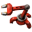Posts: 81
Joined: Sun Oct 29, 2017 7:05 pm
Contact:

### Re: Version 0.16.50

Liono2010 wrote:I am not sure what does this mean.
Your assumption "You are looking at everything in the form of binary" is wrong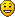pleegwat
Fast Inserter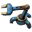Posts: 162
Joined: Fri May 19, 2017 7:31 pm
Contact:

### Re: Version 0.16.50

Liono2010 wrote:
Merssedes wrote:
Liono2010 wrote:
Merssedes wrote:(2-2).(2^(2^2)).((2+2*2*2)^2)/2WOW! That was really a nice calculation. You are looking at everything in the form of binary(2). Are you from Matrix?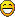Wrong assumption leads to wrong answerI am not sure what does this mean.
(2-2)=0
(2^(2^2))=2^4=16
((2+2*2*2)^2)/2=((2+8)^2)/2=(10^2)/2=100/2=50

Liono2010
Inserter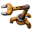Posts: 24
Joined: Mon Nov 17, 2014 9:06 pm
Contact:

### Re: Version 0.16.50

Merssedes wrote:
Liono2010 wrote:I am not sure what does this mean.
Your assumption "You are looking at everything in the form of binary" is wrongOh, okay I got that. But I saw only "2" in the expression so said the word "binary", even I know binary doesn't have the digit two.
pleegwat wrote:
Liono2010 wrote:
Merssedes wrote:
Liono2010 wrote:
Merssedes wrote:(2-2).(2^(2^2)).((2+2*2*2)^2)/2WOW! That was really a nice calculation. You are looking at everything in the form of binary(2). Are you from Matrix?Wrong assumption leads to wrong answerI am not sure what does this mean.
(2-2)=0
(2^(2^2))=2^4=16
((2+2*2*2)^2)/2=((2+8)^2)/2=(10^2)/2=100/2=50
This wasn't the thing I was asking about. However Merssedes already explained me.

### Who is online

Users browsing this forum: No registered users